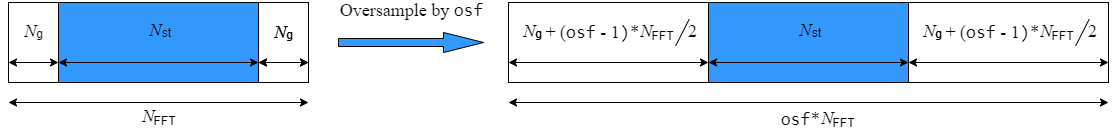# wlanSampleRate

Nominal sample rate

## Syntax

``fs = wlanSampleRate(cfgFormat)``
``fs = wlanSampleRate(cbw)``
``fs = wlanSampleRate(___,OversamplingFactor=osf)``

## Description

example

````fs = wlanSampleRate(cfgFormat)` returns the nominal sample rate for the specified WLAN transmission parameters.```

example

````fs = wlanSampleRate(cbw)` returns the nominal sample rate for the specified channel bandwidth.```

example

````fs = wlanSampleRate(___,OversamplingFactor=osf)` returns the nominal sample for the specified oversampling factor. For more information about oversampling, see FFT-Based Oversampling.```

## Examples

collapse all

Get the sample rate for a WLAN wake-up radio (WUR) transmission.

```cfgWUR = wlanWURConfig; fs = wlanSampleRate(cfgWUR)```
```fs = 20000000 ```

Get the sample rate for WLAN transmissions with specified bandwidths.

Specify a channel bandwidth of 160 MHz

`cbw = 'CBW160';`

Return the sample rate.

`fs = wlanSampleRate(cbw)`
```fs = 160000000 ```

Get the sample rate for an oversampled high-efficiency trigger-based (HE TB) WLAN transmission.

```cfgHETB = wlanHETBConfig; osf = 2.5; fs = wlanSampleRate(cfgHETB,Oversamplingfactor=osf)```
```fs = 50000000 ```

## Input Arguments

collapse all

Channel bandwidth, specified as one of these values.

• `'CBW1'` — Channel bandwidth of 1 MHz

• `'CBW2'` — Channel bandwidth of 2 MHz

• `'CBW4'` — Channel bandwidth of 4 MHz

• `'CBW5'` — Channel bandwidth of 5 MHz

• `'CBW8'` — Channel bandwidth of 8 MHz

• `'CBW10'` — Channel bandwidth of 10 MHz

• `'CBW16'` — Channel bandwidth of 16 MHz

• `'CBW20'` — Channel bandwidth of 20 MHz

• `'CBW40'` — Channel bandwidth of 40 MHz

• `'CBW80'` — Channel bandwidth of 80 MHz

• `'CBW160'` — Channel bandwidth of 160 MHz

• `'CBW320'` — Channel bandwidth of 320 MHz

Data Types: `char` | `string`

Oversampling factor, specified as a scalar greater than or equal to 1.

Data Types: `single` | `double` | `int8` | `int16` | `int32` | `int64` | `uint8` | `uint16` | `uint32` | `uint64`

## Output Arguments

collapse all

Sample rate, in samples per second, returned as a scalar.

## Algorithms

collapse all

### FFT-Based Oversampling

An oversampled signal is a signal sampled at a frequency that is higher than the Nyquist rate. WLAN signals maximize occupied bandwidth by using small guardbands, which can pose problems for anti-imaging and anti-aliasing filters. Oversampling increases the guardband width relative to the total signal bandwidth, thereby increasing the number of samples in the signal.

This function performs oversampling by using a larger IFFT and zero pad when generating an OFDM waveform. This diagram shows the oversampling process for an OFDM waveform with NFFT subcarriers comprising Ng guardband subcarriers on either side of Nst occupied bandwidth subcarriers.## Version History

Introduced in R2017b

expand all